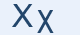Greek Letter Khi (Χι - Χ)The Greeks were the first to invent this letter. The deep-throated sound of this letter is not used in English.

"Χ" Letter in mathematics and in science

χ represents:

• the chi distribution in statistics (X2 is the more frequently encountered chi-square distribution)
• the chromatic number of a graph in graph theory
• the Euler characteristic in algebraic topology
• a variable in algebraic equations
• electronegativity in the periodic table
• the Rabi frequency
• the Fourier transform of a linear response function

Greek words with "χ"

χώμα, χαρτί